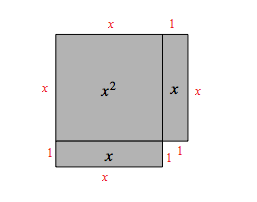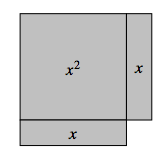### Home > MC2 > Chapter 3 > Lesson 3.2.5 > Problem3-108

3-108.

Which expressions are equivalent to the perimeter of the shape? How do you know?

1. $x+3+3x+1$

1. $2x+4+x$

1. $4x+4$

1. $2x+2+2x+2$

$x^2\text{-tiles}$ have a width of $x$ and a length of $x$.
$x\text{-tiles}$ have a width of $1$ and a length of $x$.

Since the perimeter is the distance around a shape, we must find the lengths of all the sides of the shape. Using the hint given above, we can fill in the lengths around the entire shape.Circle all of the expressions that show the sum of all the lengths of the shape.

Note: Some expressions may have combined terms differently.